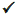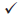# IBDP Maths AI: Topic : SL 1.5: Laws of exponents: IB style Questions SL Paper 1

## Question

Five pipes labelled, “6 metres in length”, were delivered to a building site. The contractor measured each pipe to check its length (in metres) and recorded the following;

5.96, 5.95, 6.02, 5.95, 5.99.

(i) Find the mean of the contractor’s measurements.

(ii) Calculate the percentage error between the mean and the stated, approximate length of 6 metres.


a.

Calculate $$\sqrt {{{3.87}^5} – {{8.73}^{ – 0.5}}}$$, giving your answer

(i) correct to the nearest integer,

(ii) in the form $$a \times 10^k$$, where 1 ≤ a < 10, $$k \in {\mathbb{Z}}$$ .


b.

## Markscheme

(i) Mean = (5.96 + 5.95 + 6.02 + 5.95 + 5.99) / 5 = 5.974 (5.97)     (A1)

(ii) $${\text{% error}} = \frac{{error}}{{actualvalue}} \times 100\%$$

$$= \frac{{6 – 5.974}}{{5.974}} \times 100\% = 0.435\%$$     (M1)(A1)(ft)

(M1) for correctly substituted formula.

Allow 0.503% as follow through from 5.97

Note: An answer of 0.433% is incorrect.     (C3)

[3 marks]

a.

number is 29.45728613

(i) Nearest integer = 29     (A1)

(ii) Standard form = 2.95 × 101 (accept 2.9 × 101)     (A1)(ft)(A1)

Award (A1) for each correct term

Award (A1)(A0) for 2.95 × 10     (C3)

[3 marks]

b.

## Question

Write down the following numbers in increasing order.

$$3.5$$, $$1.6 \times 10^{−19}$$, $$60730$$, $$6.073 \times 10^{5}$$, $$0.006073 \times 10^6$$, $$\pi$$, $$9.8 \times 10^{−18}$$.


a.

Write down the median of the numbers in part (a).


b.

State which of the numbers in part (a) is irrational.


c.

## Markscheme

$$1.6 \times 10^{−19}$$, $$9.8 \times 10^{−18}$$, $$\pi$$, $$3.5$$, $$0.006073 \times 10^6$$, $$60730$$, $$6.073 \times 10^{5}$$     (A4)

Award (A1) for $$\pi$$ before 3.5

Award (A1) for $$1.6 \times 10^{−19}$$ before $$9.8 \times 10^{−18}$$

Award (A1) for the three numbers containing 6073 in the correct order.

Award (A1) for the pair with negative indices placed before 3.5 and $$\pi$$ and the remaining three numbers placed after (independently of the other three marks).

Award (A3) for numbers given in correct decreasing order.

Award (A2) for decreasing order with at most 1 error     (C4)

[3 marks]

a.

The median is 3.5.     (A1)(ft)

Follow through from candidate’s list.     (C1)

[1 mark]

b.

$$\pi$$ is irrational.     (A1)     (C1)

[1 mark]

c.

## Question

Consider the numbers $$2$$, $$\sqrt 3$$, $$– \frac{2}{3}$$ and the sets $$\mathbb{N}$$, $$\mathbb{Z}$$, $$\mathbb{Q}$$ and $$\mathbb{R}$$.

Complete the table below by placing a tick in the appropriate box if the number is an element of the set, and a cross if it is not.a.

A function $$f$$ is given by $$f(x) = 2{x^2} – 3x{\text{, }}x \in \{ – 2{\text{, }}2{\text{, }}3\}$$.

Write down the range of function $$f$$.


b.

## Markscheme(A1)(A1)(A1)     (C3)

Note: Accept any symbol for ticks. Do not penalise if the other boxes are left blank.

[3 marks]

a.

$${\text{Range}} = \{ 2{\text{, }}9{\text{, }}14\}$$     (A1)(ft)     (C1)

Note: Brackets not required.

[1 mark]

b.

## Question

Consider the numbers $$\sqrt 3$$, $$6$$, $$2\frac{1}{2}$$, $$\pi$$, $$– 5$$, and the sets $$\mathbb{N}$$, $$\mathbb{Z}$$, and $$\mathbb{Q}$$. Complete the following table by placing a tick in the appropriate box if the number is an element of the set.## MarkschemeNote: Accept any symbol for ticks. Do not penalize if candidate had also indicated, by a different symbol, that the number is not an element of the set.

Row $$\mathbb{N}$$ correct, no extra entries.     (A1)     (C1)

Row $$\mathbb{Z}$$     (A1)(A1)    (C2)

Note: Award (A1) for each correct tick and no extra entries. Award (A1) only for both ticks correct and 1 extra entry, (A0) otherwise.

Row $$\mathbb{Q}$$     (A1)(A1)(A1)     (C3)

Note: Award (A1) for each correct tick and no extra entries. Award (A2) only for all 3 correct and one extra entry. Award (A1) only for 2 correct and one extra entry. (A0) otherwise.

[6 marks]

## Question

The Venn diagram shows the number sets $$\mathbb{N}$$, $$\mathbb{Z}$$, $$\mathbb{Q}$$ and $$\mathbb{R}$$. Place each of the following numbers in the appropriate region of the Venn diagram.

$$\frac{{1}}{{4}}$$, −3, π, cos 120°, 2.7 × 103, 3.4 × 10–2## Markscheme(A1)(A1)(A1)(A1)(A1)(A1)     (C6)

Note: Award (A1) for each number placed once in the correct section. Accept equivalent forms for numbers.

[6 marks]

## Question

The sets $$P$$, $$Q$$ and $$U$$ are defined as

U = {Real Numbers} ,  P = {Positive Numbers} and  Q = {Rational Numbers}.Write down in the correct region on the Venn diagram the numbers

$$\frac{{22}}{7}$$ ,  $$5 \times {10^{ – 2}}$$ ,  $$\sin (60^\circ )$$ ,  $$0$$ ,  $$\sqrt{{ – 8}}$$ ,  $$– \pi$$.

## Markscheme(A1)(A1)(A1)(A1)(A1)(A1)     (C6)

Note: Award (A1) for each number placed once in the correct region. Accept equivalent forms for numbers.

[6 marks]

## Question

$$U$$ is the set of all the positive integers less than or equal to $$12$$.
$$A$$ , $$B$$ and $$C$$ are subsets of $$U$$ .
$A = \{ 1{\text{, }}2{\text{, }}3{\text{, }}4{\text{, }}6{\text{, }}12\}$$B = \{ {\text{odd integers}}\}$$C = \{ 5{\text{, }}6{\text{, }}8\}$

Write down the number of elements in $$A \cap C$$ .


a.

List the elements of $$B$$ .


b.

Complete the following Venn diagram with all the elements of $$U$$ .c.

## Markscheme

$$1$$ (one)     (A1)     (C1)

Note: $$6$$, $$\{6\}$$ or $$\{1\}$$ earns no marks.

[1 mark]

a.

$$1$$, $$3$$, $$5$$, $$7$$, $$9$$, $$11$$     (A1)     (C1)

Note: Do not penalise if braces, parentheses or brackets are seen.

[1 mark]

b.(A1)(A1)(ft)(A1)(ft)(A1)(ft)     (C4)

Notes: Award (A1) for the empty set $$A \cap B \cap C$$ .

Award (A1)(ft) for the correct placement of $$6$$, $$5$$, $$1$$ and $$3$$.

Award (A1)(ft) for the correct placement of $$2$$, $$4$$, $$12$$, $$7$$, $$9$$, $$11$$, $$8$$.

Award (A1)(ft) for the correct placement of $$10$$.

[4 marks]

c.

## Question

Consider c = 5200 and d = 0.0000037.

Write down the value of r = c × d.


a.

Write down your value of r in the form a × 10k, where 1 ≤ a < 10 and $$k \in \mathbb{Z}$$.


b.

Consider the following statements about c, d and r. Only three of these statements are true.

Circle the true statements.c.

## Markscheme

r = 0.01924     (A1)     (C1)

Note: Accept 0.0192 and 0.019

[1 mark]

a.

r = 1.924 × 10−2     (A1)(ft)(A1)(ft)     (C2)

Notes: Award (A1) for 1.924, (A1) for 10−2. Accept 1.92 and 1.9. Follow through from their part (a).

[2 marks]

b.(A1)(A1)(A1)     (C3)

Notes: Award (A1) for each true statement circled. Do not follow through from part (a). Award (A1)(A1)(A0) if 1 extra term seen. Award (A1)(A0)(A0) if 2 extra terms seen. Award (A0)(A0)(A0) if all terms circled. Accept other indications of the correct statements i.e. highlighted or ticks.

[3 marks]

c.

## Question

$$U = \{ x|x{\text{ is an integer, }}2 < x < 10\}$$

A and B are subsets of U such that A = {multiples of 3}, B = {factors of 24}.

List the elements of

(i) U ;

(ii) B .


a.

Write down the elements of U on the Venn diagram.b.

Write down $$n (A \cap B)$$.


c.

## Markscheme

(i) 3, 4, 5, 6, 7, 8, 9     (A1)

(ii) 3, 4, 6, 8     (A1)(ft)     (C2)

Notes: Follow through from part (a)(i).

[2 marks]

a.(A1)(ft) for their 3, 6
(A1)(ft) for their 4, 8, 9
(A1)(ft) for their 5, 7     (A1)(ft)(A1)(ft)(A1)(ft)     (C3)

Note: Follow through from their universal set and set B in part (a).

[3 marks]

b.

2     (A1)(ft)     (C1)

Note: Follow through from their Venn diagram.

[1 mark]

c.

## Question

Consider the numbers 3, −5 , $$\sqrt{7}$$, $$2^{−3}$$ and 1.75.

Complete the table below, placing a tick () to show which of the number sets, $$\mathbb{N}, \mathbb{Q} {\text{ and }} \mathbb{R}$$ these numbers belong to. The first row has been completed as an example.## Markscheme(A1) for $$\mathbb{N}$$ column correct.

(A2) for $$\mathbb{R}$$ column correct, award (A1) if oneis missing, award (A0) if two or moremissing.

(A3) for $$\mathbb{Q}$$ column correct, award (A2) for two correctly placedand no extra entries, award (A1) for one correctly placedand no extra entries orplaced in all entries.     (A6)     (C6)

[N/A]

## Question

The following Venn diagram shows the relationship between the sets of numbers

$\mathbb{N},{\text{ }}\mathbb{Z}{\text{, }}\mathbb{Q}{\text{ and }}\mathbb{R}{\text{.}}$

The number –3 belongs to the set of $$\mathbb{Z}{\text{, }}\mathbb{Q}$$ and $$\mathbb{R}$$,  but not $$\mathbb{N}$$, and is placed in the appropriate position on the Venn diagram as an example.Write down the following numbers in the appropriate place in the Venn diagram.

a. 4 

b. $$\frac{1}{3}$$

c. $$\pi$$
d. $$0.38$$
e. $$\sqrt 5$$
f. $$-0.25$$

## Markscheme(A1)(A1)(A1)(A1)(A1)(A1)     (C6)

Note: Award (A1) for each number correctly placed.

Award (A0) for any entry in more than one region.

[1 mark]

a.(A1)(A1)(A1)(A1)(A1)(A1)     (C6)

Note: Award (A1) for each number correctly placed.

Award (A0) for any entry in more than one region.

[1 mark]

b.(A1)(A1)(A1)(A1)(A1)(A1)     (C6)

Note: Award (A1) for each number correctly placed.

Award (A0) for any entry in more than one region.

[1 mark]

c.(A1)(A1)(A1)(A1)(A1)(A1)     (C6)

Note: Award (A1) for each number correctly placed.

Award (A0) for any entry in more than one region.

[1 mark]

d.(A1)(A1)(A1)(A1)(A1)(A1)     (C6)

Note: Award (A1) for each number correctly placed.

Award (A0) for any entry in more than one region.

[1 mark]

e.(A1)(A1)(A1)(A1)(A1)(A1)     (C6)

Note: Award (A1) for each number correctly placed.

Award (A0) for any entry in more than one region.

[1 mark]

f.

## Question

Consider the numbers $$– 1,\,\,4,\,\,\frac{2}{3},\,\,\sqrt 2 ,\,\,0.35$$ and $$– {2^2}$$.

Complete the following table by placing a tick () to indicate if the number is an element of the number set. The first row has been completed as an example.## Markscheme(A1)(A1)(A1)(A1)(A2)     (C6)

Note: Row 1 has been given in the question.
Row 2 to row 5: Award (A1) for each correct row.
Row 6: Award (A1) for both $$\mathbb{N}$$ not selected and $$\mathbb{Z}$$ selected; award (A1) for both $$\mathbb{Q}$$ and $$\mathbb{R}$$ selected. Do not penalize if crosses (or similar) appear in the empty cells.

## Question

The following table shows four different sets of numbers: $$\mathbb{N}$$, $$\mathbb{Z}$$, $$\mathbb{Q}$$ and $$\mathbb{R}$$.Complete the second column of the table by giving one example of a number from each set.


a.

Josh states: “Every integer is a natural number”.


b.

## Markscheme(A1)(A1)(A1)(A1)(C4)

[4 marks]

a.

Incorrect     (A1)

Natural numbers are positive integers. Integers can also be negative. (or equivalent)     (R1) (C2)

Note: Accept a correct justification. Do not award (R0)(A1).
Accept: a statement with an example of an integer which is not natural.

[2 marks]

b.